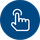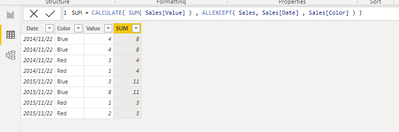cancel
Showing results for
Did you mean:Helper II

## Calculated column with sum as function

I'm looking for a formula that uses sums as logic that we know in Excel. I want to add a calculated column (orange) in the table below. That sums values of column value if the column values of date and color are equal.I once came across a formula with the EARLIER function, but I don't know the exact formula to get it to work.

1 ACCEPTED SOLUTIONSuper User

Hint: You should never use EARLIER. There is a much better alternative and it's called VARIABLES.

``````// This is the formula for your column...

[Total Value] =
var CurrentDate = YourTable[Date]
var CurrentColor = YourTable[Color]
var Output =
sumx(
filter(
YourTable,
YourTable[Date] = CurrentDate
&&
YourTable[Color] = CurrentColor
),
YourTable[Value]
)
return
Output``````

5 REPLIES 5Helper II

I want to thank you both ( @daXtreme & @zerotyper  ) for the quick and well-functioning response. I have been helped tremendously.

As a result of @daXtreme 's additional explanation, I naturally opt for his solution.

Thanks and have a nice weekend!Frequent Visitor

@brief001 Hope this is the result you want.Super User

A word of caution.

Using CALCULATE in calculated column is a no-no. This slows DAX tremendously down even on moderate sized sets. I've seen many a time formulas like this that brought the engine down to a complete freeze.Frequent Visitor

@daXtreme Really thanks for your suggestion.

You are right, just use Variable and simply filter and Aggregation is better than Calculate in a calculated column.

The context transition is too heavy.Super User

Hint: You should never use EARLIER. There is a much better alternative and it's called VARIABLES.

``````// This is the formula for your column...

[Total Value] =
var CurrentDate = YourTable[Date]
var CurrentColor = YourTable[Color]
var Output =
sumx(
filter(
YourTable,
YourTable[Date] = CurrentDate
&&
YourTable[Color] = CurrentColor
),
YourTable[Value]
)
return
Output``````